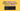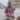# Why Math.max() Without Arguments Returns -Infinity

Posted May 18, 2021Start discussion

`Math.max()` is a built-in JavaScript utility function that determines the maximum number from the arguments.

For example, let’s determine the maximum of the numbers `1`, `2` and `3`:

`javascript`Math.max(1, 2, 3); // => 3``

As expected, `3` is the maximum of `1`, `2`, and `3`.

What would happen if `Math.max()` is invoked with just one argument:

`javascript`Math.max(1); // => 1``

As expected, the maximum of one number is the number itself.

But what would happen if you invoke `Math.max()` without arguments at all?

`javascript`Math.max(); // => -Infinity``

While it might be unexpected at first, but calling `Math.max()` without arguments returns `-Infinity`. Let’s find out why it happens, and why it’s important to happen this way.

## 1. Max of one array

Before diving into the main question (why `Math.max()` without args returns `-Infinity`), let’s see how `Math.max()` can be used to determine the maximum number from an array.

`Math.max(num1, num2, ..., numN)` accepts multiple number arguments and returns the maximum number of them. Simple as a pie.

If you want to determine the maximum number of an array, then you can use the spread arguments operator on the array:

`javascript`const numbers1 = [1, 2, 3];Math.max(...numbers1); // => 3``

`Math.max(...numbers1)` expression uses the spread syntax and returns the maximum number of `numbers1` array: `3`.

## 2. Max of 2 arrays

Now let’s try something more interesting. Given two arrays of numbers, let’s determine the maximum number of each array, and then determine the maximum of these 2 maximum values.

A bit overwhelming, but bear with me…

`javascript`const numbers1 = [1, 2, 3];const numbers2 = [0, 6];const max1 = Math.max(...numbers1);const max2 = Math.max(...numbers2);max1; // 3max2; // 6Math.max(max1, max2); // => 6``

The maximum number of `[1, 2, 3]` is `3`, and of `[0, 6]` is corresponding `6`. The max value from `3` and `6` is `6`.

That’s expected.

What about trying to determine the maximum of arrays if one array is empty? Let’s make `numbers1` an empty array and try again:

`javascript`const numbers1 = [];const numbers2 = [0, 6];const max1 = Math.max(...numbers1);const max2 = Math.max(...numbers2);max1; // -Infinitymax2; // 6Math.max(max1, max2); // => 6``

Now, having the first array empty, the above code snippet correctly determines `6` as being the maximum of 2 arrays.

What’s interesting is what value returns `Math.max(...numbers1)`: being that `numbers1` array is empty, this is the same as calling `Math.max()` without arguments. Correspondingly, this is `-Infinity`.

Then `Math.max(max1, max2)` is evaluated as `Math.max(-Infinity, 6)`, which results in `6`.

Now it’s clear why `Math.max()` returns `-Infinity` when called without arguments: it’s a way for the max function to be defined upon an empty set.

Making a parallel between max and addition, `-Infinity` for max is the same as `0` for addition.

The same behavior happens with `Math.min()` — it returns `Infinity` when called without arguments.

`-Infinity`, in regards to the max operation on the real numbers, is called the Identity element.

## 3. Conclusion

`Math.max()` called without arguments returns `-Infinity` to correctly handle the cases multiple maximum operations are performed (max of max of max…), particularly being useful when determining the max value of an empty array.

In simple words, if you’re determining the maximum numbers of 2 arrays, and then determine the maximum of these maximums too, you would get the expected result.

`javascript`Math.max(  Math.max(...[]),    // -Infinity  Math.max(...[0, 6]) // 6); // => 6``

Challenge: Can you write a `sum(num1, num2, ..., numN)` function that works exactly like `Math.max()`, only that it summarizes its argument? What would be the identity element? Share your solution in a comment below!

## Quality posts into your inbox

I regularly publish posts containing:

• Important JavaScript concepts explained in simple words
• Overview of new JavaScript features
• How to use TypeScript and typing
• Software design and good coding practices